# Initialization and Accessing Pointers in C Programming

We have so far seen how to declare different types of pointers. Let us see how to initialize them – make them point to address of another variable, and how to access them.

Suppose intX is a normal variable of type integer and intPtrX is a pointer variable pointing to intX. If intPtrX has to point to address of intX, then we have to initialize intPtrX to the address of intX. Address of any variable is obtained by adding ‘&’ (address operator) before the variable name.

&intX = BD0023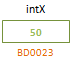Hence initializing intPtrX would be as below.

intPtrX = &intX; // this assigns address of intX to intPtrX.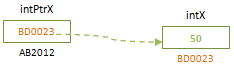Now pointer variable intPtrX contains the address of intX as shown above. Suppose now we want to access the data value that is present in intX using the pointer. Then we use ‘*’ before the pointer variable name to get the value of the variable that it is pointing to. The ‘*’ operator is known as dereferencing operator.

printf (“Value of intX is %d”, *intPtrX); // Value of intX is 50

When a pointer variable used without dereferencing operator, it gives the address that is stored in it (content of pointer variable). When it is referred with address operator before it, it gives its own address.

```printf (“The address of y is: %d\n”, &intPtrX); // The address of intPtrX is: AB2012
printf (“The address stored at y is: %d\n”, intPtrX); // The address stored at intPtrX is: BD0023
printf (“The value of *y (as a pointer) is: %d\n”, *intPtrX); // The value of *intPtrX (as a pointer) is: 50```

From above example, it is clear that pointer variable acts same as any other normal variable with only difference when dereferencing operator is used.

If we want to change the value of variable, intX, we can directly assign the value to *intPtrX.

*intPtrX = 100; // this is same as assigning intX = 100;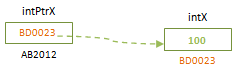See below set of operations and observe how they are changing the values at different steps.

```#include <stdio.h>
int main()
{
int   intX, intY;
int   *intPtrX;
intX = 25;
intPtrX = &intX; // intPtrX points to intX
intY = *intPtrX; // intY gets the value that intPtrX is pointing to  = 25
intPtrX = &intY; // pointer intPtrX is changed to point to intY
*intPtrX = 60;   // intY value is changed to 60
return 0;
}```

See below the diagrammatic representation of above code at different steps to understand it better.

intPtrX = &intX; //intPtrX points to intX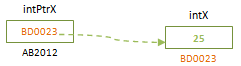intY = *intPtrX; //intY gets the value that intPtrX is pointing to = 25: Observe below that pointer is not pointing to intY; only the value that intPtrX pointing is copied to intY.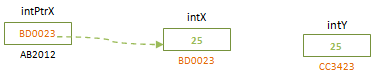intPtrX = &intY; // pointer intPtrX is changed to point to intY: Now the pointer intPtrX has address of intY and it is not pointing to intX now.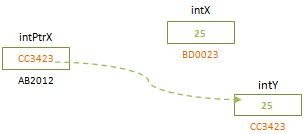*intPtrX = 60; // intY value is changed to 60: Value if intY is changed to 60 since the pointer variable intPtrX is now pointing to intY. The value of intX remains unchanged.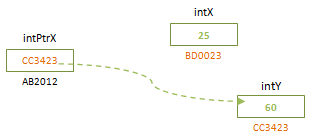We can initialize and access float, double, character pointers in the same way as above.

```flPtrY = &fltY; // Initializing a float pointer
dblPtrS = &dblS; // Initializing a double pointer
chrPtrZ = &chrZ; // Initializing a character pointer
*flPtrY = 3.14; // Assigning the value to a float pointer; hence to the float variable that it is pointing to.```

When structure pointers are used in a program, then its elements are initialized and dereferenced as below.

```#include <stdio.h>
struct structExample {
int intX;
char chrY;
};

int main()
{
Struct structExample structX;
struct structExample *structPtr;
char chrZ;

structPtr = &structX; // Points to the structure structX – initializing structure pointer
*structPtr.intX = 25; // Assigning values to intX of structX
structPtr->chrY = ‘C’; // Another method of assigning value

chrZ = *structPtr.chrZ; // Assigning the value of structure element to character variable
return 0;
}```

In addition to using ‘*’ as dereferencing operator, in structures we can use ‘→’ as dereferencing operator. If we are using ‘→’ with pointers, then ‘*’ is not used before the pointer variable.

Translate »